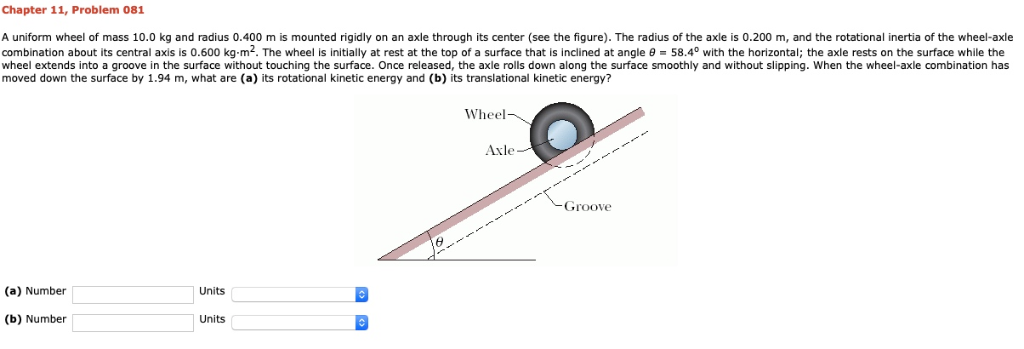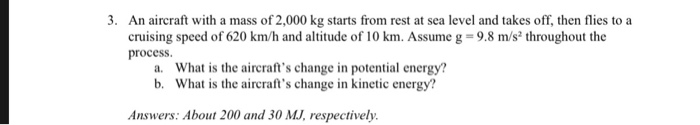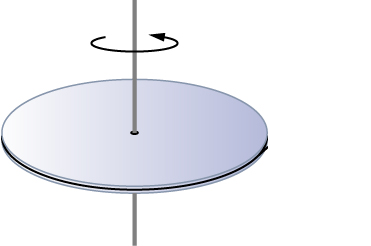Question

An automobile has a total mass of 1100 kg. It accelerates from rest to 69 km/h in 12 s. Assume each wheel is a uniform 23 kg disk. Find, for the end of the 12 s interval, (a) the rotational kinetic energy of each wheel about its axle, (b) the total kinetic energy of each wheel, and (c) the total kinetic energy of the automobile.

(A) Rotational Kinetic Energy : Kr = (1/2)*I*(w^2)

I for the disc about its (1/2)*m*R^2

Kr = (1/2)*(1/2)*m*R^2*(w^2) = (1/4)*m*v^2 = (1/4)*23*(69*1000/60*60)^2 = 2112.3 J

(B) Kinetic energy = Kv = (1/2)*m*v^2 = (1/2)*23*(69*1000/60*60)^2 = 4224.6 J

So total KE = Kr + Kv = 6336.9 J

(C) Total Kinetic energy = (1/2)*m*V^2 = (1/2)*1100*(69*1000/60*60) ^2

= 202048.6 J

#### Earn Coins

Coins can be redeemed for fabulous gifts.

Similar Homework Help Questions
• ### Chapter 11, Problem 081 A uniform wheel of mass 10.0 kg and radius 0.400 m is...Chapter 11, Problem 081 A uniform wheel of mass 10.0 kg and radius 0.400 m is mounted rigidly on an axle through its center (see the figure). The radius of the axle is 0.200 m, and the rotational inertia of the wheel-axle combination about its central axis is 0.600 kg-m2. The wheel is initially at rest at the top of a surface that is inclined at angle 58.4° with the horizontal; the axle rests on the surface while the wheel...

• ### A uniform wheel of mass 10.0 kg and radius 0.400 m is mounted rigidly on an axle through its center

A uniform wheel of mass 10.0 kg and radius 0.400 m is mounted rigidly on an axle through its center (see figure . The radius of the axle is 0.200 m, and the rotational inertia of the wheel-axle combination about its central axis is 0.600 kg·m2. The wheel is initially at rest at the top of a surface that is inclined at angleθ = 43.6o with the horizontal; the axle rests on the surface while the wheel extends into a...

• ### An automobile has a mass of 1200 kg. What is its kinetic energy, in kJ, relative...

An automobile has a mass of 1200 kg. What is its kinetic energy, in kJ, relative to the road when traveling at a velocity of 50 km/h? If the vehicle accelerates to 100 km/h in 15 seconds, what is the change in power required?

• ### A 1000-kg car is accelerated from rest to 85 km/h in 10 s. Assuming that no...

A 1000-kg car is accelerated from rest to 85 km/h in 10 s. Assuming that no mass is lost and the car accelerates on a flat, horizontal surface, what is the change of the total energy of the car? How much power is required to change this total energy? Would the total energy change and power required change if it were to accelerate in 5 s?

• ### A yo-yo has a rotational inertia of 1100 g·cm2 and a mass of 118 g. Its...

A yo-yo has a rotational inertia of 1100 g·cm2 and a mass of 118 g. Its axle radius is 1.99 mm, and its string is 133 cm long. The yo-yo rolls from rest down to the end of the string. (a) What is the magnitude of its linear acceleration? (b) How long does it take to reach the end of the string? As it reaches the end of the string, what are its (c) linear speed, (d) translational kinetic energy,...

• ### A 2700-kg Ferris wheel accelerates from rest to an angular speed of 0.16 rad/s in 14...

A 2700-kg Ferris wheel accelerates from rest to an angular speed of 0.16 rad/s in 14 s . Approximate the Ferris wheel as a circular disk with a radius of 23 m . What is the net torque on the wheel? Net Torque = _____ m*N

• ### . An aircraft with a mass of 2,000 kg starts from rest at sea level and.... An aircraft with a mass of 2,000 kg starts from rest at sea level and takes off, then flies to a cruising speed of 620 km/h and altitude of 10 km. Assume g-9.8 m/s throughout the process What is the aircraft's change in potential energy? What is the aircraft's change in kinetic energy? a. b. Answers: About 200 and 30 MJ respectively

• ### A 1700 kg sports car accelerates from rest to 95 km/h in 7.9 s .What is...

A 1700 kg sports car accelerates from rest to 95 km/h in 7.9 s .What is the average power delivered by the engine? Express using two significant figures.

• ### The mass of the disk is 0.35 kg with a radius of 0.18 m and is...The mass of the disk is 0.35 kg with a radius of 0.18 m and is initially spinning at 5 revolutions per second. It is spinning horizontally and on a very low friction axle. Then a uniform thin rod of length 0.40m and mass of 0.085 kg is suspended just above the spinning disk and dropped, without any rotational motion, onto the spinning disk exactly across its diameter. After the rod is dropped, the rod and disk are moving with...

• ### A carnival merry-go-round has a large disk-shaped platform of mass 120 kg that can rotate about...

A carnival merry-go-round has a large disk-shaped platform of mass 120 kg that can rotate about a center axle. A 60- kg student stands at rest at the edge of the platform 4.0 m from its center. The platform is also at rest. The student starts running clockwise around the edge of the platform and attains a speed of 2.4 m/s relative to the ground. A) Determine the rotational velocity of the platform. B) Determine the change of kinetic energy...